# Multiplier

Multiplier

In mathematics, to multiply means to add a number to itself a particular number of times.

Multiplication can be viewed as a process of repeated addition.

For example, $$4 \times 3$$ is the same as $$3+3+3+3=12$$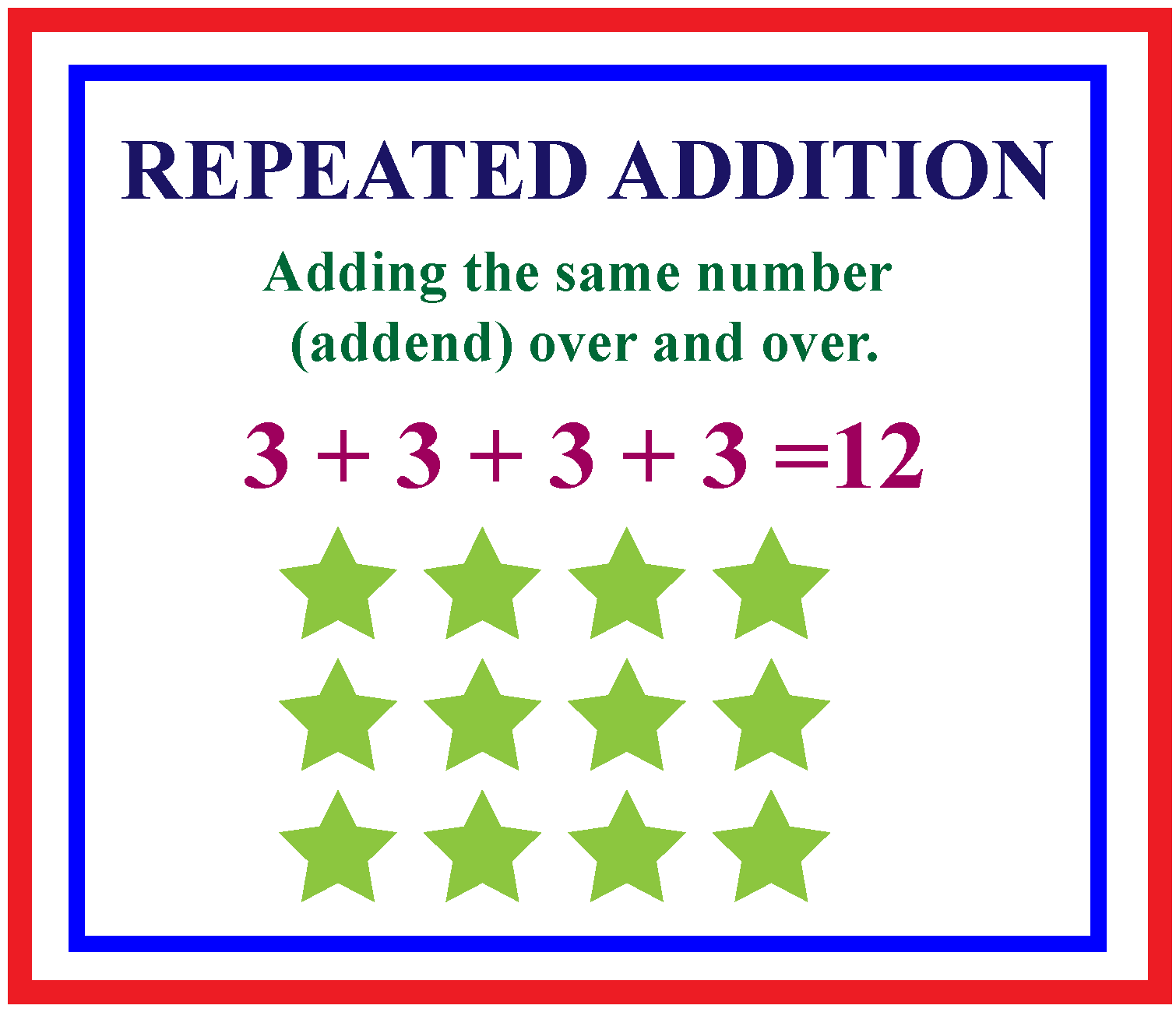In this short lesson, we will learn about the multiplier and the multiplicand.

## Lesson Plan

 1 What Is Meant by Multiplier? 2 Important Notes on Multiplier 3 Solved Examples on Multiplier 4 Thinking out of the Box! 5 Interactive Questions on Multiplier

## What Is Meant by Multiplier?

### Meaning of Multiplier in Math

Look at the 3 groups of 7 cupcakes.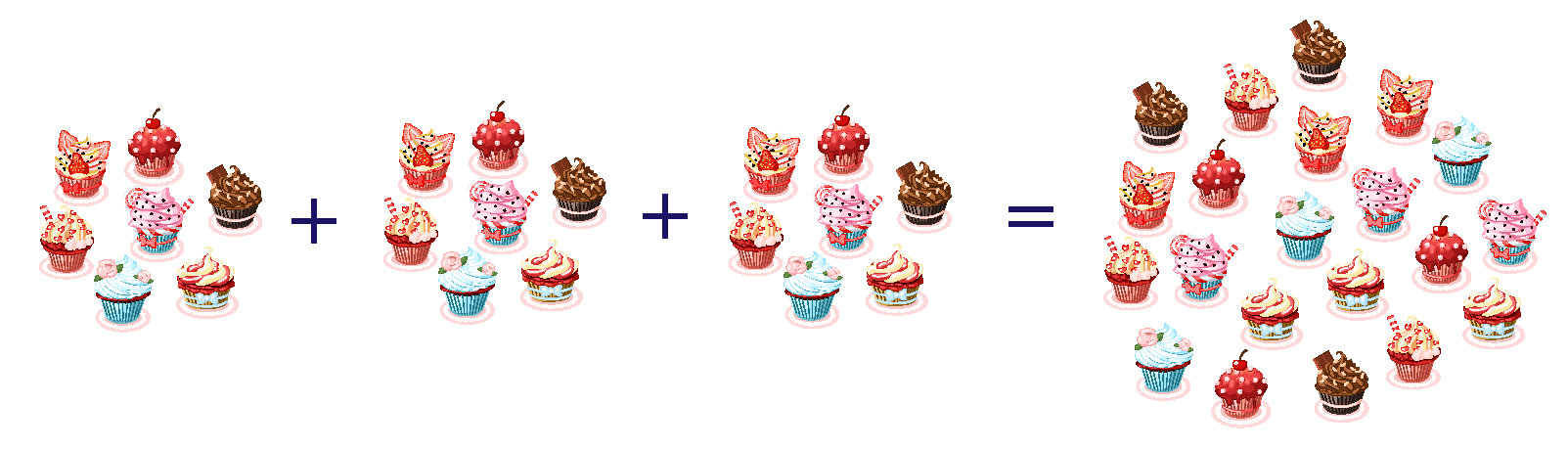Instead of adding seven three times, we can write it as $$7 \times 3 =21$$

This means there are 21 cupcakes in all.

Here, 7 is the multiplier and 3 is the multiplicand.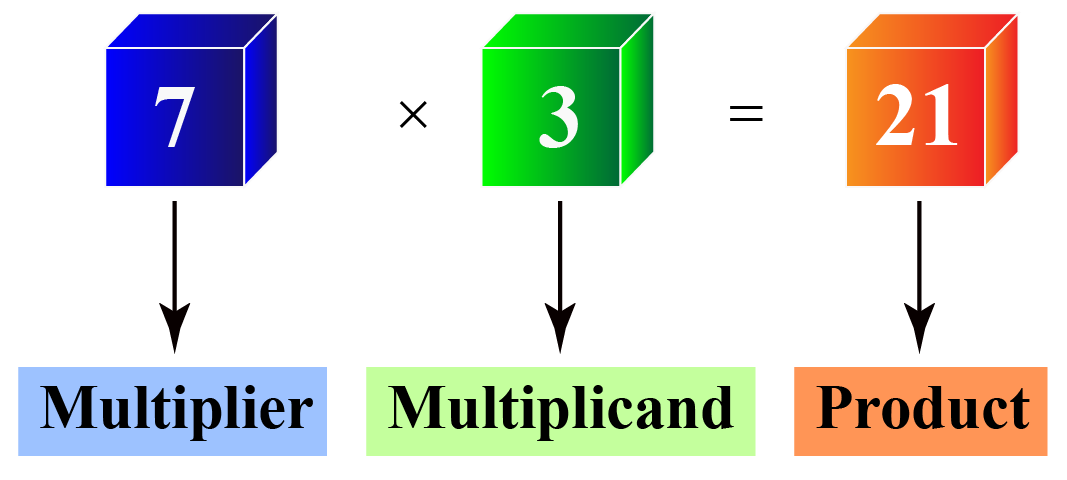Multiplier and multiplicand are parts of a multiplication statement.

## How To Find a Product Using Multiplier?

When we write a multiplication statement in a horizontal way, the leftmost number is the multiplier.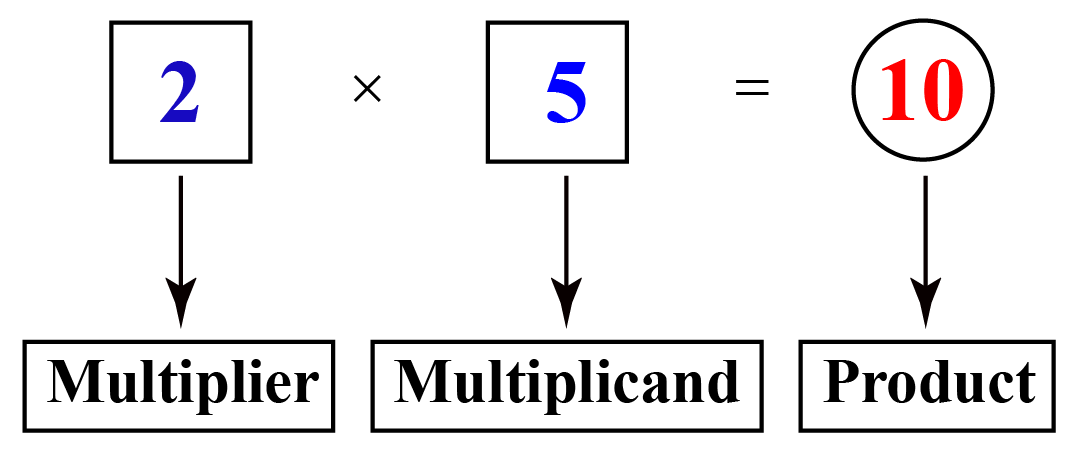When we write a multiplication statement in a vertical way, the number on the top is the multiplier.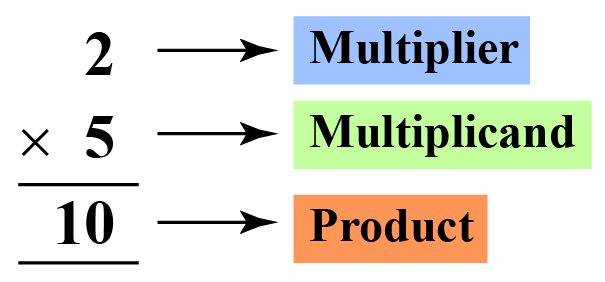Look at the grid shown below.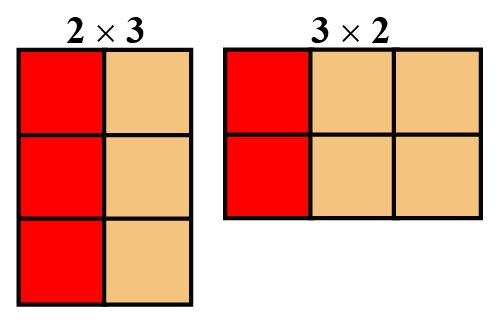All the boxes contained in the grid correspond to the product of multiplier and multiplicand.

This is one of the easiest methods used for numbers with smaller quantities.

Experiment with the simulation below by using sliders to change multiplier and multiplicand and observe how the product changes.

## Solved Examples

 Example 1

Tom got 3 packs of trading cards and each pack had 5 cards in it.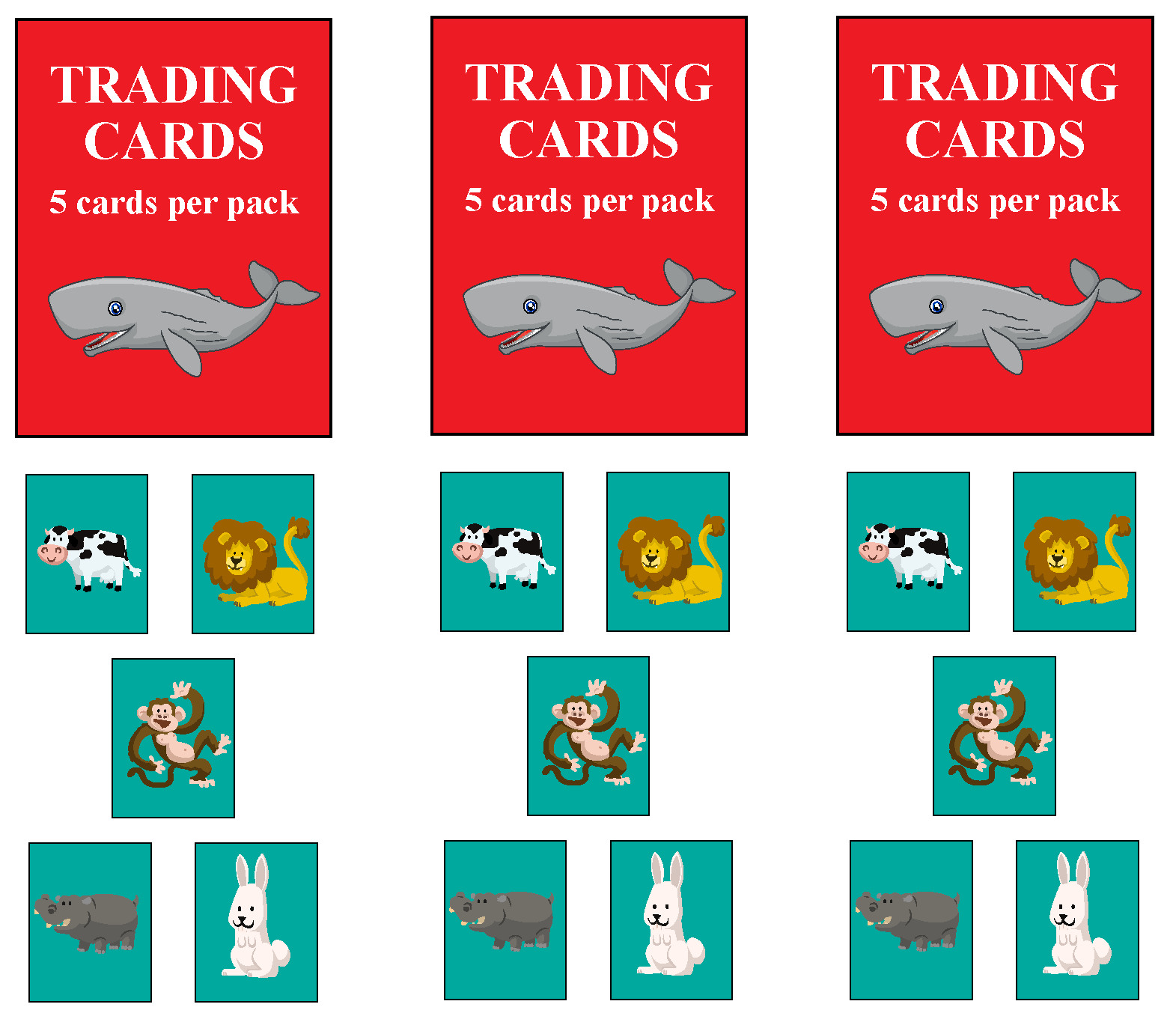So, how many cards did Tom get in total?

Express this as a multiplication statement and state the multiplier and the multiplicand.

### Solution

Number of cards in one pack of trading cards = 5

Number of cards in 3 packs of trading cards = $$5 \times 3=15$$

So, Tom got 15 cards in all.

 The multiplier and the multiplicand are 5 and 3 respectively.
 Example 2

A frog has to jump on every third tile without stepping on any number in between.

It starts jumping from the number 3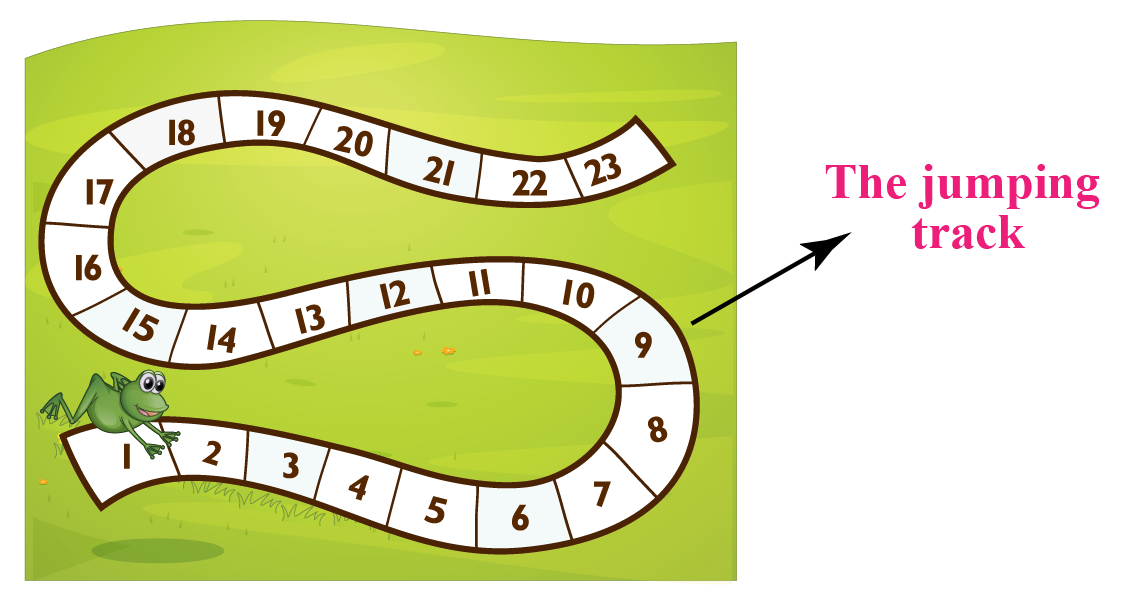Can you list the tiles that he jumps on?

What is the multiplier here?

Solution

Let us color each 3rd tile as the frog jumps on the track.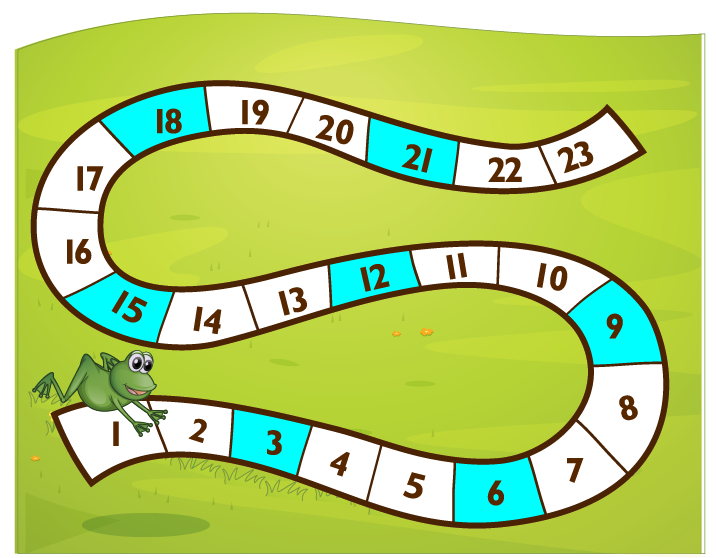We observe that the tiles that the frog jumps on are multiples of 3: 3, 6, 9, 12, 15, 18,...

 So, the multiplier is 3Think Tank
 1. The following card contains an incomplete 5-column grid.  Do appropriate calculations to find the missing numbers. Finally, there are five selected numbers on each grid. Transfer them to the final column, and the last challenge is to find the sum all of these numbers.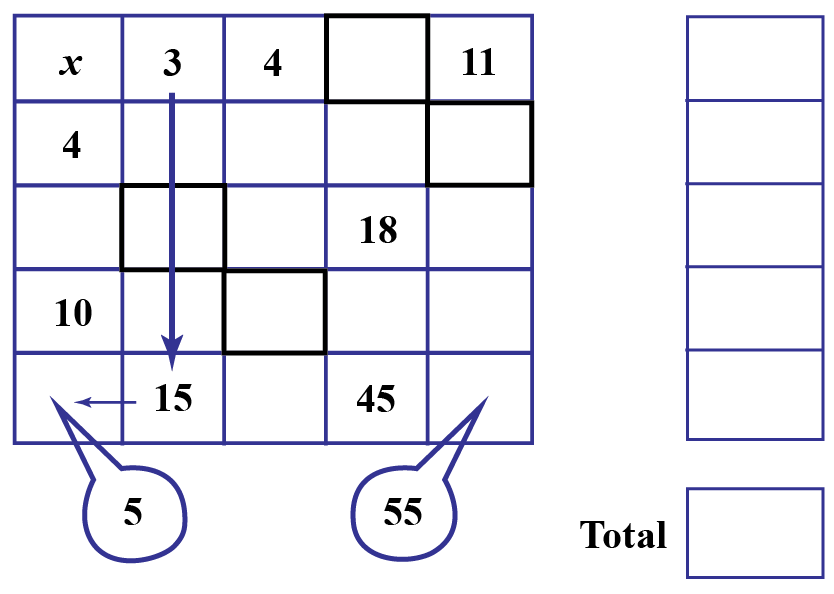## Interactive Questions

Here are a few activities for you to practice.

## Let's Summarize

This mini-lesson targeted the fascinating concept of Multiplier. The math journey around Multiplier starts with what a student already knows, and goes on to creatively crafting a fresh concept in the young minds. Done in a way that is not only relatable and easy to grasp, but will also stay with them forever. Here lies the magic with Cuemath.

We hope you enjoyed learning about the meaning of multiplier in math.

At Cuemath, our team of math experts is dedicated to making learning fun for our favorite readers, the students!

Through an interactive and engaging learning-teaching-learning approach, the teachers explore all angles of a topic.

Be it worksheets, online classes, doubt sessions, or any other form of relation, it’s the logical thinking and smart learning approach that we, at Cuemath, believe in.

## 1. Why is the multiplier important?

The multiplier is important because it is a factor that amplifies the base value of the given quantity.

## 2. Is a multiplier always greater than 1?

No, the multiplier can take the value 1

More Important Topics
Numbers
Algebra
Geometry
Measurement
Money
Data
Trigonometry
Calculus
More Important Topics
Numbers
Algebra
Geometry
Measurement
Money
Data
Trigonometry
Calculus
Learn from the best math teachers and top your exams

• Live one on one classroom and doubt clearing
• Practice worksheets in and after class for conceptual clarity
• Personalized curriculum to keep up with school